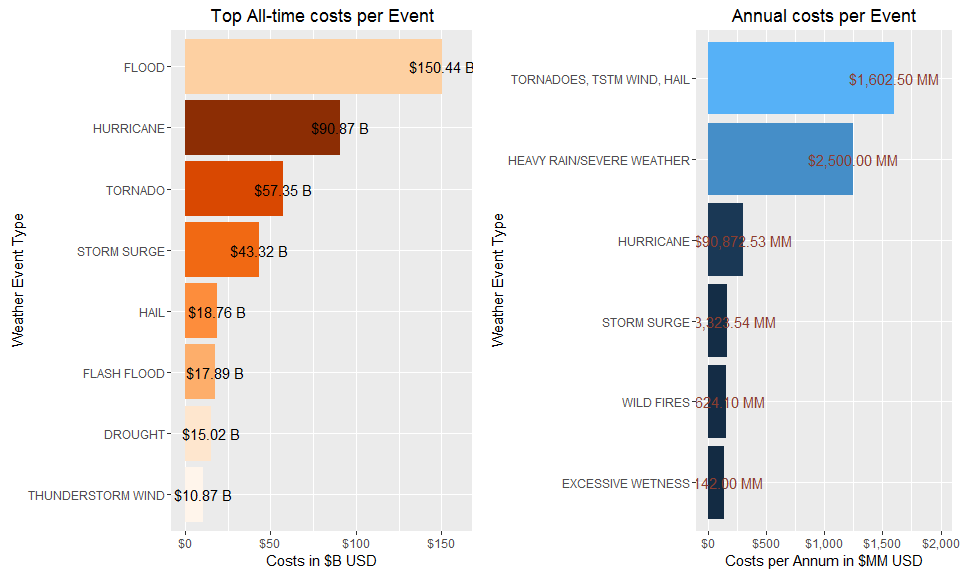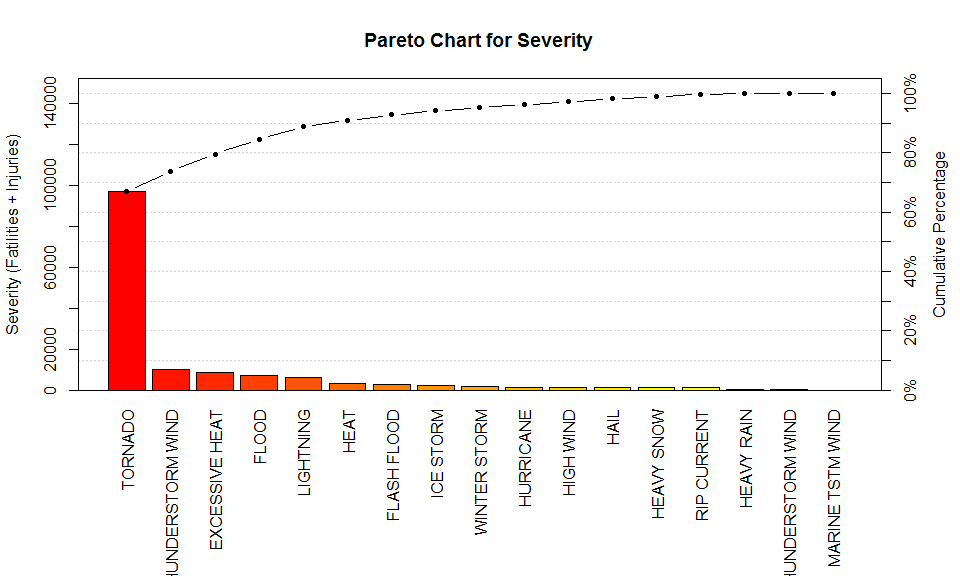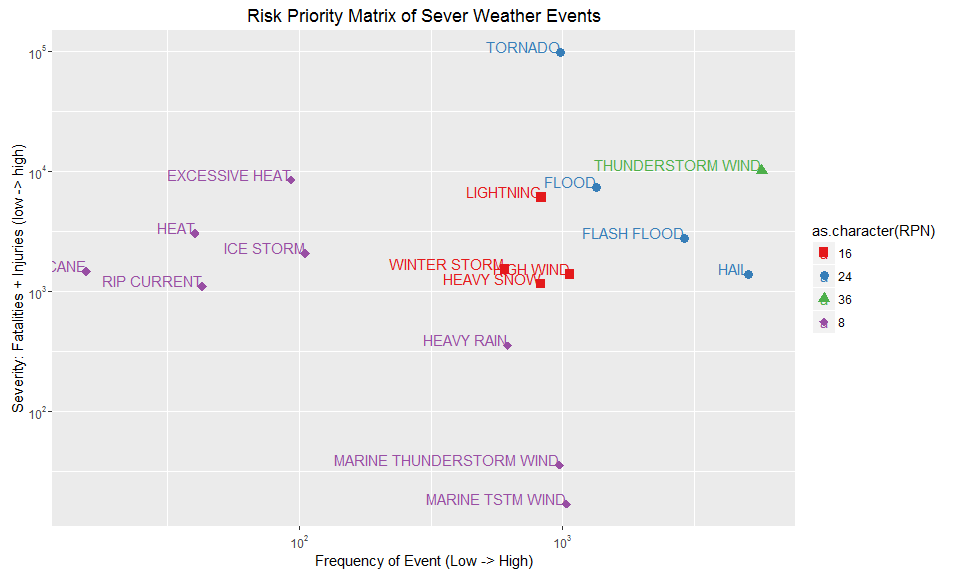## Synopsis

This report helps communities plan for impacts from severe weather events. It will prioritize severe weather events in terms of safety and economic impact. Events are categorized into a risk priority ranking based on severity and frequency. Severity is a measure of the impact on fatalities and injuries. Frequency is how often the event is likely to occur. Events triggering high economic impact to the community both overall and per annum are also presented.

Over the 62 years between 1950 and 2011, the riskiest event to human safety are Tornados causing 96,979 fatalities and injuries. Floods caused the most economic damage at $150.4 billion. The mostly costly events per annum also include tornadoes and thunderstorm winds at$1.6B

The Results section will explain how these determinations are made and show where the remainder of events fall in priority.

## Data Processing

The following packages were used to perform this analysis…

setwd("C:/Users/Mike/Documents/Projects/dataScience/ReproducibleResearch/Assignment 2")
library(dplyr)
library(qcc)
library(ggplot2)
library(scales)
library(gridExtra)

The data is downloaded from the course web site

Storm data documentation can be found at the National Weather Service web site

# Download, unpack, and load data into a data frame...
download.file("https://d396qusza40orc.cloudfront.net/repdata%2Fdata%2FStormData.csv.bz2", "StormData.csv.bz2")
stormData <- read.csv("StormData.csv.bz2")

# Add a column indicating the year of the event (all events have a beginning date)
stormData$year <- as.numeric(format(as.Date(stormData$BGN_DATE, "%m/%d/%Y"), format="%Y"))

First, convert the economic impact fields of property and crop damage into numbers. The PROPDMGEXP and CROPDMGEXP fields indicate magnitude such that, “K” for thousands, “M” for millions, and “B” for billions.

Many of the magnitude fields are missing or have unexpected values. In these cases, the cost will be $0 because there is no accurate way to determine the intended value. The total economic impact (crop + property) is stored in the TotalEconomicDMG variable. # Convert property magnitude fields to numbers stormData$PROPDMGEXP <- ifelse(stormData$PROPDMGEXP =="K", 1000, ifelse(stormData$PROPDMGEXP == "m" | stormData$PROPDMGEXP == "M", 1000000,ifelse(stormData$PROPDMGEXP =="B", 1000000000,0)))

# Add a column to hold the property damage amount...
stormData$PropDMGValue <- stormData$PROPDMG * stormData$PROPDMGEXP # Do the same for crop damage... stormData$CROPDMGEXP <- ifelse(stormData$CROPDMGEXP =="K" | stormData$CROPDMGEXP =="k", 1000, ifelse(stormData$CROPDMGEXP == "m" | stormData$CROPDMGEXP == "M", 1000000,ifelse(stormData$CROPDMGEXP =="B", 1000000000,0))) stormData$CropDMGValue <- stormData$CROPDMG * stormData$CROPDMGEXP

# Now, add up the total economic damage...
stormData$TotalEconomicDMG <- stormData$CropDMGValue + stormData$PropDMGValue The total number of fatalities + injuries will determine the severity of an event # To determine severity of events, add a column to show the injuries + fatalities stormData$HumanDMG <- stormData$FATALITIES + stormData$INJURIES

Some event types seem to have different names for the same event, for example, these event types could be the same: TSTM WIND, THUNDERSTORM WIND, and THUNDERSTORM WINDS

To find the meaningful event type inconsistencies, I sorted a list of event types and counts over 100. The following event types have been ‘cleaned’:

stormData$EVTYPE[stormData$EVTYPE == "COASTAL FLOODING"] <- "COASTAL FLOOD"
stormData$EVTYPE[stormData$EVTYPE == "FLASH FLOODING"] <- "FLASH FLOOD"
stormData$EVTYPE[stormData$EVTYPE == "FLASH FLOODS"] <- "FLASH FLOOD"
stormData$EVTYPE[stormData$EVTYPE == "FLASH FLOOD/FLOOD"] <- "FLOOD/FLASH FLOOD"
stormData$EVTYPE[stormData$EVTYPE == "FLOODING"] <- "FLOOD"
stormData$EVTYPE[stormData$EVTYPE == "FUNNEL CLOUD"] <- "FUNNEL"
stormData$EVTYPE[stormData$EVTYPE == "FUNNEL CLOUDS"] <- "FUNNEL"
stormData$EVTYPE[stormData$EVTYPE == "Gusty Wind"] <- "GUSTY WINDS"
stormData$EVTYPE[stormData$EVTYPE == "HEAVY RAINS"] <- "HEAVY RAIN"
stormData$EVTYPE[stormData$EVTYPE == "HURRICANE/TYPHOON"] <- "HURRICANE"
stormData$EVTYPE[stormData$EVTYPE == "TYPHOON"] <- "HURRICANE"
stormData$EVTYPE[stormData$EVTYPE == "LAKE-EFFECT SNOW"] <- "LAKE EFFECT SNOW"
stormData$EVTYPE[stormData$EVTYPE == "RIP CURRENTS"] <- "RIP CURRENT"
stormData$EVTYPE[stormData$EVTYPE == "RIVER FLOODING"] <- "RIVER FLOOD"
stormData$EVTYPE[stormData$EVTYPE == "STRONG WINDS"] <- "STRONG WIND"
stormData$EVTYPE[stormData$EVTYPE == "THUNDERSTORM WINDS"] <- "THUNDERSTORM WIND"
stormData$EVTYPE[stormData$EVTYPE == "THUNDERSTORM WINDSS"] <- "THUNDERSTORM WIND"
stormData$EVTYPE[stormData$EVTYPE == "THUNDERSTORMS WINDS"] <- "THUNDERSTORM WIND"
stormData$EVTYPE[stormData$EVTYPE == "TSTM WIND"] <- "THUNDERSTORM WIND"
stormData$EVTYPE[stormData$EVTYPE == "WATERSPOUTS"] <- "WATERSPOUT"
stormData$EVTYPE[stormData$EVTYPE == "WINDS"] <- "WIND"

stormData$EVTYPE[grep("HURRICANE", stormData$EVTYPE)] <- "HURRICANE"

Now, create a summarized table of events where each row is an event type and the other components are quantified as such,

• yearspan - The span of years for which each event has been reported - used to determine frequency of events
• EventCount - the number of occurances of this event in the dataset
• Severity - the sum of fatalities and injuries for that event
• Costs - the sum of all economic costs (crop + prop)
# Group by event and perform calculations on each field...
EVStats <- group_by(stormData, EVTYPE) %>% summarize(yearSpan = (max(year) - min(year))+1, EVCount = NROW(EVTYPE), Severity = as.integer(sum(HumanDMG)), Costs = sum(TotalEconomicDMG)) %>% arrange(desc(Costs))

Calculate the frequency in terms of events per year and classify into three categories,

• highly frequent
• Moderately frequent
• and rare
# Determine frequency of event by determining occurrence per year...
EVStats$Frequency <- EVStats$EVCount / EVStats$yearSpan EVStats$annCosts <- EVStats$Costs / EVStats$EVCount

# Classify frequency into three categories, High(6) Frequency, Medium Frequency(4), low Frequency(2)
EVStats$FrequencyClass <- ifelse(EVStats$Frequency > 1200, 6, ifelse(EVStats$Frequency < 500, 2, 4)) # Classify Severity into three categories, High(6), Medium(4), low(2) EVStats$SeverityClass <- ifelse(EVStats$Severity > 10000, 6, ifelse(EVStats$Severity < 1000, 2, 4))

Calculate a Risk Priority Number by multiplying severity * frequency

# Calculate the Risk Priority Number...
EVStats$RPN <- EVStats$FrequencyClass * EVStats$SeverityClass Prepare the data to show event severity in a pareto chart # Prepare data for the pareto.chart function... PCRPN <- EVStats[EVStats$RPN > 4,]$Severity names(PCRPN) <- EVStats[EVStats$RPN > 4,]$EVTYPE Prepare a Risk Priority scatter plot (using log10 scales) # A scatter plot of RPN RPNScatter <- ggplot(EVStats[EVStats$RPN > 4,], aes(x=Frequency, y=Severity, color=as.character(RPN), shape = as.character(RPN))) + geom_point() + geom_point(size=3) + scale_shape_manual(values=c(15,16,17,18,19)) + scale_colour_brewer(palette="Set1") + geom_text(aes(label=EVTYPE), size=4, vjust=0, hjust=1) + ggtitle("Risk Priority Matrix of Sever Weather Events") + xlab("Frequency of Event (Low -> High)") + ylab("Severity: Fatalities + Injuries (low -> high)") + labs(fill="Risk Priority Number (RPN)") + scale_x_log10(breaks=10^(0:10), labels=trans_format("log10", math_format(10^.x))) + scale_y_log10(breaks=10^(0:10), labels=trans_format("log10", math_format(10^.x)))

Prepare economic cost charts

# Economic Costs Bar Plots
allCostChart <- ggplot(EVStats[EVStats$Costs > 10158548500, ], aes(x=reorder(EVTYPE, Costs), y=Costs/1000000000, fill = as.character(Costs))) + geom_bar(stat='identity') + coord_flip() + geom_text(aes(label=paste(dollar(Costs/1000000000), "B")), hjust=.5, vjust=.5, colour="black", position=position_dodge(.9), size=4) + scale_fill_brewer(palette="Oranges") + labs(y = "Costs in$B USD", x = "Weather Event Type") + scale_y_continuous(labels=dollar, limits = c(0, 160)) + guides(fill = FALSE) + ggtitle("Top All-time costs per Event")

allCostChartYearly <- ggplot(EVStats[EVStats$annCosts > 100000000, ], aes(x=reorder(EVTYPE, annCosts), y=annCosts/1000000, fill=annCosts)) + geom_bar(stat='identity') + coord_flip() + geom_text(aes(label=paste(dollar(Costs/1000000), "MM")), hjust=.5, vjust=.5, colour="coral4", position=position_dodge(.9), size=4) + labs(y = "Costs per Annum in$MM USD", x = "Weather Event Type") + scale_y_continuous(labels=dollar, limits = c(0, 2000)) + guides(fill = FALSE) + ggtitle("Annual costs per Event")

## Results

Presented below are the costliest events in terms of economic impact and human safety

### Economic Damage

grid.arrange(allCostChart, allCostChartYearly, ncol=2)Figure 1: Ranking Economic Costs

Flood Hurricanes, and tornadoes are the top three most expensive events throughout the 62-year study period. You can see that similar events are also the costliest annually. These events are costly and occur more frequently.

### Severity

The Pareto analysis below ranks the events from most severe (measured by fatalities + injuries) to least and also shows the relative magnitude thereof.

pareto.chart(PCRPN, cumperc = seq(0, 100, by = 10), ylab = "Severity (Fatilities + Injuries)", main="Pareto Chart for Severity")Figure 2: Ranking Human Cost

##
## Pareto chart analysis for PCRPN
##                            Frequency Cum.Freq.  Percentage Cum.Percent.
##   TORNADO                      96979     96979 66.84841425     66.84841
##   THUNDERSTORM WIND            10059    107038  6.93375059     73.78216
##   EXCESSIVE HEAT                8428    115466  5.80948902     79.59165
##   FLOOD                         7267    122733  5.00920226     84.60086
##   LIGHTNING                     6046    128779  4.16755702     88.76841
##   HEAT                          3037    131816  2.09342883     90.86184
##   FLASH FLOOD                   2784    134600  1.91903387     92.78088
##   ICE STORM                     2064    136664  1.42273200     94.20361
##   WINTER STORM                  1527    138191  1.05257353     95.25618
##   HURRICANE                     1466    139657  1.01052574     96.26671
##   HIGH WIND                     1385    141042  0.95469178     97.22140
##   HAIL                          1376    142418  0.94848800     98.16989
##   HEAVY SNOW                    1148    143566  0.79132575     98.96121
##   RIP CURRENT                   1101    144667  0.75892826     99.72014
##   HEAVY RAIN                     353    145020  0.24332577     99.96347
##   MARINE THUNDERSTORM WIND        36    145056  0.02481509     99.98828
##   MARINE TSTM WIND                17    145073  0.01171824    100.00000

80% of fatalities and injuries result from three types of weather events, tornado, thunderstorm wind, and excessive heat.

### Risk Priority Ranking

print(RPNScatter)Figure 3: Frequency and Severity

This plot shows the relationship between the frequency and severity of recorded events. Thunderstorm Winds are a big threat because they cause high damage (fatalities and injuries) and occur frequently. Tornados, while being the most severe events occur less frequently. The Risk Priority Number (RPN) ranks the events that pose the biggest threat to safety. The ranking considers both factors and in general means:

• RPN of 36 is the riskiest events
• RPN of 24 are highly risky events
• RPN of 16 are moderately risky
• RPN of 8 are least risky

The top five riskiest weather related events to human safety are,

1. Thunderstorm Winds
2. Tornados
3. Floods
4. Flash Floods
5. Hail